# Dirac Notation for Vectors and Tensors (Neuenschwander's text ....)

• I
• Math Amateur
A \rangle = \begin{bmatrix} A^1 \\ A^2 \\ A^3 \end{bmatrix} ##which is what I was hoping to achieve.

#### Math Amateur

Gold Member
MHB
I am reading Tensor Calculus for Physics by Dwight E. Neuenschwander and am having difficulties in confidently interpreting his use of Dirac Notation in Section 1.9 ...

in Section 1.9 we read the following: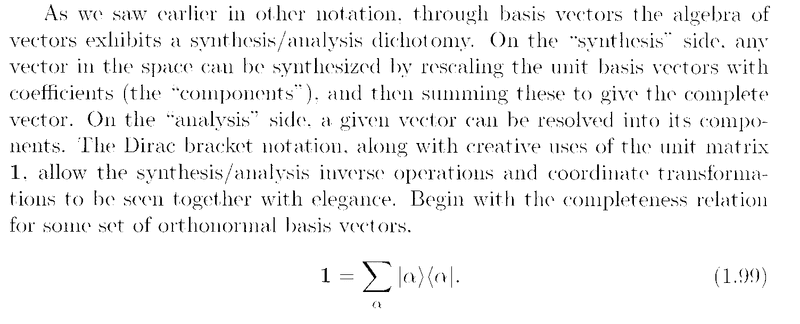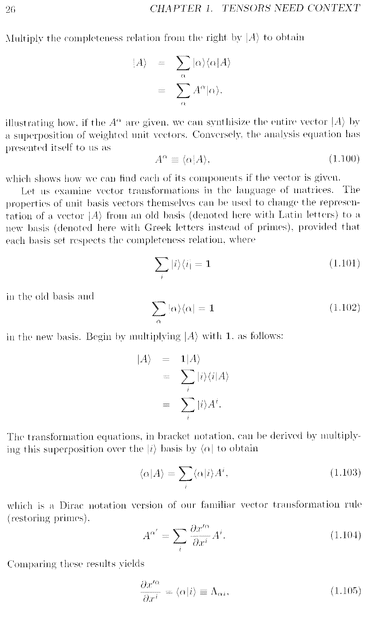I need some help to confidently interpret and proceed with Neuenschwander's notation in the text above ...

Indeed I am not sure how to interpret Neuenschwander when he writes:

## 1 = \sum_{ \alpha } \vert \alpha \rangle \langle \alpha \vert ##... ... ... (1.99)

Am I proceeding validly or correctly when i assume that

## | \alpha \rangle =
\begin{bmatrix}
\alpha^1 \\
\alpha^2 \\
\alpha^3
\end{bmatrix}

##

... and

## \langle \alpha | = \begin{bmatrix}
\alpha^1 & \alpha^2 & \alpha^3
\end{bmatrix}
##

and when he writes

##\vert A \rangle = \sum_{ \alpha } \vert \alpha \rangle \langle \alpha \vert A \rangle ##

... can I assume that it is OK to take

## \vert A \rangle = \begin{bmatrix} A^1 \\ A^2 \\ A^3 \end{bmatrix} ##

so that

## \langle \alpha \vert A \rangle =

\begin{bmatrix}
\alpha^1 & \alpha^2 & \alpha^3
\end{bmatrix}

\begin{bmatrix}
A^1 \\
A^2 \\
A^3
\end{bmatrix}
##

... and so on ...

Am i proceeding correctly ?

Hope someone can help ...

Peter

#### Attachments

Last edited:
Hello Peter.
Broadly you have the correct idea but your approach relies on two implicit assumptions that do not hold in the general case.
Firstly, by writing ##\langle \alpha|## and ##|\alpha\rangle## as each having three components, you are assuming the vector space is finite dimensional. Dirac's notation is most used in quantum mechanics, where the vector spaces are usually infinite-dimensional, so such a representation as a finite set of components does not appear.
Secondly, you replace the inner product ##\langle\alpha|A\rangle## by a simple multiplication of a row vector ##\langle\alpha|## by a column vector ##|A\rangle##. That is only correct when those vectors are representations in an orthonormal basis. From your first embedded image, that seems to be the case here, but you need to exercise care that it will not always be the case. The more general formula requires use of a metric tensor so that ##\langle\alpha|A\rangle = \langle\alpha| \times M \times |\alpha\rangle## where ##M## is the representation of the metric tensor in the given basis. For an orthonormal basis ##M## will be the identity matrix (with an infinite number of rows and columns if the space is infinite-dimensional!) so the calculation collapses to look like what you have shown.
Lastly, to avoid confusion, avoid writing things like $$\vert A \rangle = \begin{bmatrix} A^1 \\ A^2 \\ A^3 \end{bmatrix}$$
The left-hand side is a vector in the space. The right-hand side is a representation of that vector in a particular basis. The two sides are not the same sort of object and should not be connected by an equals sign.

This can seem confusing if you've been introduced to linear algebra through vectors and matrices that are rows, columns or rectangle of numbers. Vector spaces are abstract objects, of which numeric number tuples are only one type.

•Math Amateur and topsquark

I very much appreciate your help …

Thanks again,

Peter

andrewkirk said:
Hello Peter.
Broadly you have the correct idea but your approach relies on two implicit assumptions that do not hold in the general case.
Firstly, by writing ##\langle \alpha|## and ##|\alpha\rangle## as each having three components, you are assuming the vector space is finite dimensional. Dirac's notation is most used in quantum mechanics, where the vector spaces are usually infinite-dimensional, so such a representation as a finite set of components does not appear.
Secondly, you replace the inner product ##\langle\alpha|A\rangle## by a simple multiplication of a row vector ##\langle\alpha|## by a column vector ##|A\rangle##. That is only correct when those vectors are representations in an orthonormal basis. From your first embedded image, that seems to be the case here, but you need to exercise care that it will not always be the case. The more general formula requires use of a metric tensor so that ##\langle\alpha|A\rangle = \langle\alpha| \times M \times |\alpha\rangle## where ##M## is the representation of the metric tensor in the given basis. For an orthonormal basis ##M## will be the identity matrix (with an infinite number of rows and columns if the space is infinite-dimensional!) so the calculation collapses to look like what you have shown.
Lastly, to avoid confusion, avoid writing things like $$\vert A \rangle = \begin{bmatrix} A^1 \\ A^2 \\ A^3 \end{bmatrix}$$
The left-hand side is a vector in the space. The right-hand side is a representation of that vector in a particular basis. The two sides are not the same sort of object and should not be connected by an equals sign.

This can seem confusing if you've been introduced to linear algebra through vectors and matrices that are rows, columns or rectangle of numbers. Vector spaces are abstract objects, of which numeric number tuples are only one type.
Andrew,

Do you have any recommended texts on Dirac Notaation for vectors and tensors …?

Peter

The ones I have used are in Quantum Mechanics texts.
'Principles of Quantum Mechanics' by Shankar has what seemed to me a nicely-paced introduction to it.
The other text I have that covers it is 'Quantum mechanics' by Cohen-Tannoudji - a highly respected text but it's a big purchase - two heavy volumes!

••PeroK and topsquark
Thanks Andrew …

Math Amateur said:
Am I proceeding validly or correctly when i assume that

## | \alpha \rangle =
\begin{bmatrix}
\alpha^1 \\
\alpha^2 \\
\alpha^3
\end{bmatrix}

##

... and

## \langle \alpha | = \begin{bmatrix}
\alpha^1 & \alpha^2 & \alpha^3
\end{bmatrix}
##
To add to @andrewkirk answer, not that what you wrote for the bra is incorrect, it should read
## \langle \alpha | = \begin{bmatrix}
\bar{\alpha}^1 & \bar{\alpha}^2 & \bar{\alpha}^3
\end{bmatrix}
##
i.e., the vector components of the bra are the complex conjugates of those of the ket.

•Math Amateur and topsquark
andrewkirk said:
The other text I have that covers it is 'Quantum mechanics' by Cohen-Tannoudji - a highly respected text but it's a big purchase - two heavy volumes!
I love Cohen-Tannoudji! And they're actually still printing it!

-Dan

•Math Amateur
topsquark said:
I love Cohen-Tannoudji! And they're actually still printing it!

-Dan
Dan,

Does it have enough worked examples?

Does the book provide any solutions to exercises …

‘’These items can help one get a good grasp of theory …

Peter

andrewkirk said:
The ones I have used are in Quantum Mechanics texts.
'Principles of Quantum Mechanics' by Shankar has what seemed to me a nicely-paced introduction to it.
The other text I have that covers it is 'Quantum mechanics' by Cohen-Tannoudji - a highly respected text but it's a big purchase - two heavy volumes!
Andrew.

Do the Cohen-Tannoudji volumes have a good number of worked examples … ?

Does it have worked solutions to some of the exercises …?

I think these items can help one get a good grasp of the theory …

Peter

Math Amateur said:
Dan,

Does it have enough worked examples?

Does the book provide any solutions to exercises …

‘’These items can help one get a good grasp of theory …

Peter
It has a number of examples, explicitly worked out, but at that level you will find that the worked examples and the exercises don't overlap all that well. However the text is relentless in its application of Mathematics to the problem of developing Intro QM. (I'm finding as time goes on I'm leaning more and more to Mathematical Physics.) Once you have the notation down the text is very clearly organized and covers practically any loose ends you might run into.

IMHO I wouldn't say it's the best text(s) to learn from but it makes for one heck of a good review. I'm afraid I don't have any source suggestions to add to andrewkirk's post. My introduction to QM (and particularly QFT) was kind of "non-standard."

-Dan

topsquark said:
It has a number of examples, explicitly worked out, but at that level you will find that the worked examples and the exercises don't overlap all that well. However the text is relentless in its application of Mathematics to the problem of developing Intro QM. (I'm finding as time goes on I'm leaning more and more to Mathematical Physics.) Once you have the notation down the text is very clearly organized and covers practically any loose ends you might run into.

IMHO I wouldn't say it's the best text(s) to learn from but it makes for one heck of a good review. I'm afraid I don't have any source suggestions to add to andrewkirk's post. My introduction to QM (and particularly QFT) was kind of "non-standard."

-Dan
Oh … interesting …

Peter

andrewkirk said:
Hello Peter.
Broadly you have the correct idea but your approach relies on two implicit assumptions that do not hold in the general case.
Firstly, by writing ##\langle \alpha|## and ##|\alpha\rangle## as each having three components, you are assuming the vector space is finite dimensional. Dirac's notation is most used in quantum mechanics, where the vector spaces are usually infinite-dimensional, so such a representation as a finite set of components does not appear.
Secondly, you replace the inner product ##\langle\alpha|A\rangle## by a simple multiplication of a row vector ##\langle\alpha|## by a column vector ##|A\rangle##. That is only correct when those vectors are representations in an orthonormal basis. From your first embedded image, that seems to be the case here, but you need to exercise care that it will not always be the case. The more general formula requires use of a metric tensor so that ##\langle\alpha|A\rangle = \langle\alpha| \times M \times |\alpha\rangle## where ##M## is the representation of the metric tensor in the given basis. For an orthonormal basis ##M## will be the identity matrix (with an infinite number of rows and columns if the space is infinite-dimensional!) so the calculation collapses to look like what you have shown.
Lastly, to avoid confusion, avoid writing things like $$\vert A \rangle = \begin{bmatrix} A^1 \\ A^2 \\ A^3 \end{bmatrix}$$
The left-hand side is a vector in the space. The right-hand side is a representation of that vector in a particular basis. The two sides are not the same sort of object and should not be connected by an equals sign.

This can seem confusing if you've been introduced to linear algebra through vectors and matrices that are rows, columns or rectangle of numbers. Vector spaces are abstract objects, of which numeric number tuples are only one type.

andrewkirk said:
Hello Peter.
Broadly you have the correct idea but your approach relies on two implicit assumptions that do not hold in the general case.
Firstly, by writing ##\langle \alpha|## and ##|\alpha\rangle## as each having three components, you are assuming the vector space is finite dimensional. Dirac's notation is most used in quantum mechanics, where the vector spaces are usually infinite-dimensional, so such a representation as a finite set of components does not appear.
Secondly, you replace the inner product ##\langle\alpha|A\rangle## by a simple multiplication of a row vector ##\langle\alpha|## by a column vector ##|A\rangle##. That is only correct when those vectors are representations in an orthonormal basis. From your first embedded image, that seems to be the case here, but you need to exercise care that it will not always be the case. The more general formula requires use of a metric tensor so that ##\langle\alpha|A\rangle = \langle\alpha| \times M \times |\alpha\rangle## where ##M## is the representation of the metric tensor in the given basis. For an orthonormal basis ##M## will be the identity matrix (with an infinite number of rows and columns if the space is infinite-dimensional!) so the calculation collapses to look like what you have shown.
Lastly, to avoid confusion, avoid writing things like $$\vert A \rangle = \begin{bmatrix} A^1 \\ A^2 \\ A^3 \end{bmatrix}$$
The left-hand side is a vector in the space. The right-hand side is a representation of that vector in a particular basis. The two sides are not the same sort of object and should not be connected by an equals sign.

This can seem confusing if you've been introduced to linear algebra through vectors and matrices that are rows, columns or rectangle of numbers. Vector spaces are abstract objects, of which numeric number tuples are only one type.

andrewkirk said:
Hello Peter.
Broadly you have the correct idea but your approach relies on two implicit assumptions that do not hold in the general case.
Firstly, by writing ##\langle \alpha|## and ##|\alpha\rangle## as each having three components, you are assuming the vector space is finite dimensional. Dirac's notation is most used in quantum mechanics, where the vector spaces are usually infinite-dimensional, so such a representation as a finite set of components does not appear.
Secondly, you replace the inner product ##\langle\alpha|A\rangle## by a simple multiplication of a row vector ##\langle\alpha|## by a column vector ##|A\rangle##. That is only correct when those vectors are representations in an orthonormal basis. From your first embedded image, that seems to be the case here, but you need to exercise care that it will not always be the case. The more general formula requires use of a metric tensor so that ##\langle\alpha|A\rangle = \langle\alpha| \times M \times |\alpha\rangle## where ##M## is the representation of the metric tensor in the given basis. For an orthonormal basis ##M## will be the identity matrix (with an infinite number of rows and columns if the space is infinite-dimensional!) so the calculation collapses to look like what you have shown.
Lastly, to avoid confusion, avoid writing things like $$\vert A \rangle = \begin{bmatrix} A^1 \\ A^2 \\ A^3 \end{bmatrix}$$
The left-hand side is a vector in the space. The right-hand side is a representation of that vector in a particular basis. The two sides are not the same sort of object and should not be connected by an equals sign.

This can seem confusing if you've been introduced to linear algebra through vectors and matrices that are rows, columns or rectangle of numbers. Vector spaces are abstract objects, of which numeric number tuples are only one type.

Hello Andrew,

Thanks again on your help with Neuenschwander Section 1.9 ... {Note ... A scan of Neuenschwander Section 1.9 from the start to page 26 is available below ...}​
I am hoping you can clarify some issues for me ...​
You write:​
" ... ... Lastly, to avoid confusion, avoid writing things like​
## \vert A \rangle = \begin{bmatrix} A^1 \\ A^2 \\ A^3 \end{bmatrix} ##​
The left-hand side is a vector in the space. The right-hand side is a representation of that vector in a particular basis. The two sides are not the same sort of object and should not be connected by an equals sign.
... ... ... ... "

I note that the author tends to interpret the situation in 3 dimensions ... so for convenience I did the same ...

The author also says the basis ## \alpha ## is orthonormal ( see note directly before equation (1.99) [see scanned text below ... page 25 ...]

Given the context of a 3-dimension space and an orthonormal basis ... and further given the fact that we have a given basis ## \alpha ### am I justified in writing something like

## \vert A \rangle = \begin{bmatrix} A^1 \\ A^2 \\ A^3 \end{bmatrix} ##​

... see also authors equation (1.86) which has an expression similar to mine above ... [ see scanned notes below ...]

... on another issue i am completely stumped with the equations directly following equation ( 1.99 ) [ see scanned notes below ... top of page 26 ... ] ... ... that is ...

##\vert A \rangle = \sum_{ \alpha } \vert \alpha \rangle \langle \alpha \vert A \rangle ## ... ... ... (*)

In (*) all I can think in terms of spelling out the meaning is to take

## \vert A \rangle = \begin{bmatrix} A^1 \\ A^2 \\ A^3 \end{bmatrix} ##

and​
## | \alpha \rangle =
\begin{bmatrix}
\alpha^1 \\
\alpha^2 \\
\alpha^3
\end{bmatrix}

##

and

## \langle \alpha \vert A \rangle =

\begin{bmatrix}
\alpha^1 & \alpha^2 & \alpha^3
\end{bmatrix}

\begin{bmatrix}
A^1 \\
A^2 \\
A^3
\end{bmatrix}

= \alpha^1 A^1 + \alpha^2 A^2 + \alpha^3 A^3 ##

so ...

## \vert A \rangle \langle \alpha \vert A \rangle = \begin{bmatrix} \alpha^1 \\ \alpha^2 \\ \alpha^3 \end{bmatrix} [ \alpha^1 A^1 + \alpha^2 A^2 + \alpha^3 A^3 ]

= \begin{bmatrix} (\alpha^1)^2 A^1 + \alpha^1 \alpha^2 A^2 + \alpha^1 \alpha^3 A^3 \\ \alpha^2 \alpha^1 A^1 + (\alpha^2)^2 A^2 + \alpha^1 \alpha^3 A^3 \\ \alpha^3 \alpha^1 A^1 + \alpha^3 \alpha^2 A^2 + (\alpha^3)^2 A^3 \end{bmatrix} ##

... however ... why do we need the ## \sum_{ \alpha } ## in ## \sum_{ \alpha } \vert \alpha \rangle \langle \alpha \vert A \rangle ## ... and further ... how should I interpret ## \sum_{ \alpha } A^{ \alpha} \vert \alpha \rangle ##

... Is the above making sense ... ? Is the above reasonable interpretation of Neuenschwander ... ??
Hope you can help ... ?

Peter

It will be helpful if you have access to Neuenschwander Section 1.9 so i have scanned the relevant pages of Section 1.9 ... and the read as follows ...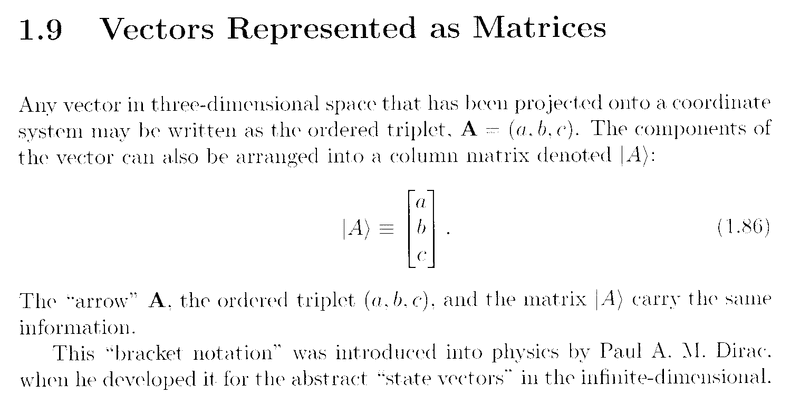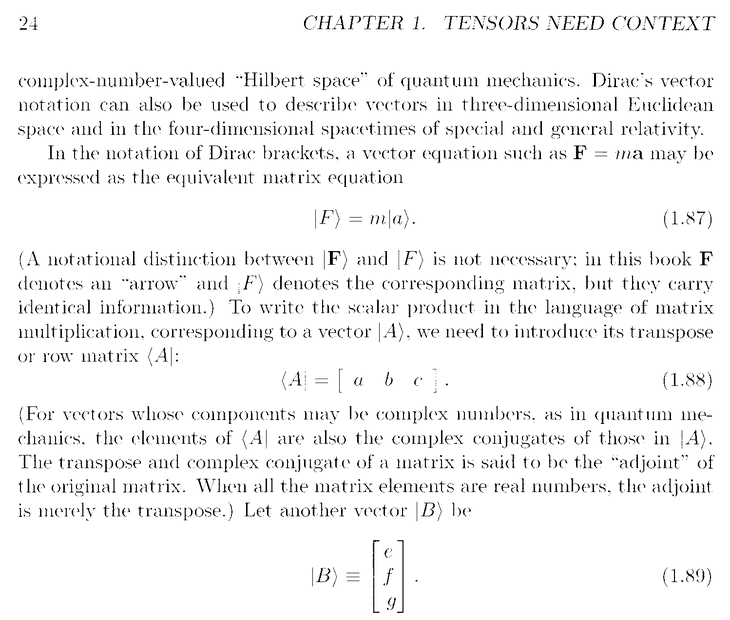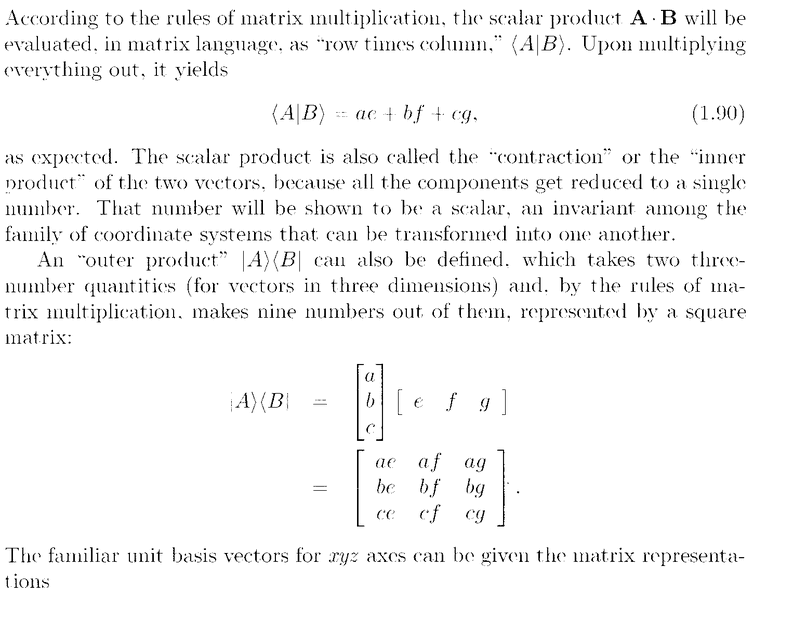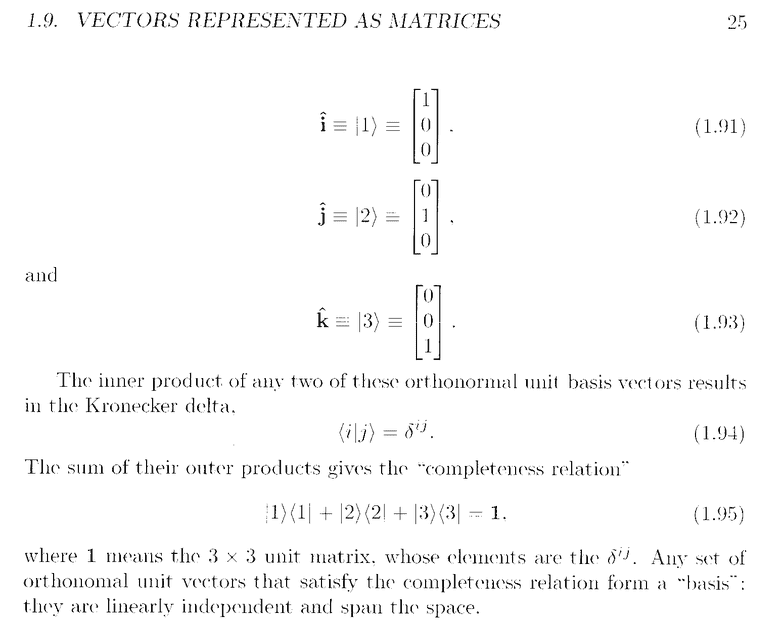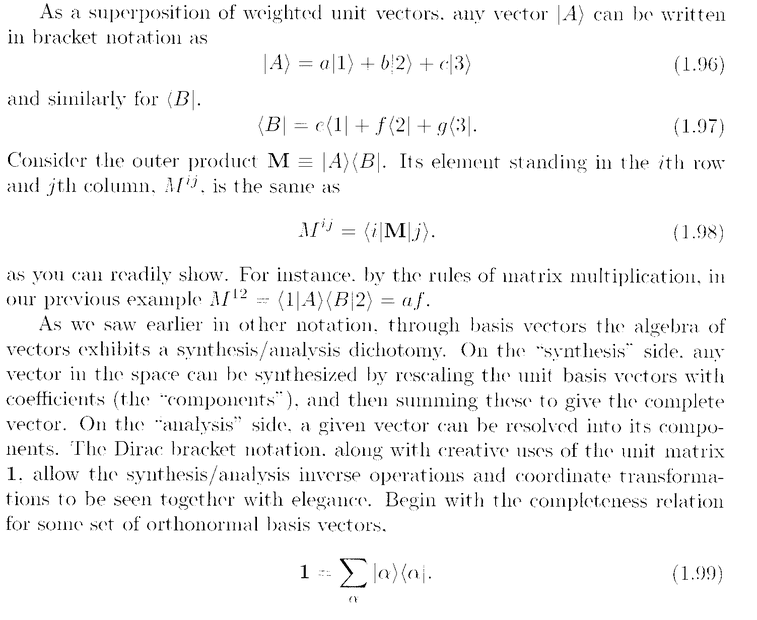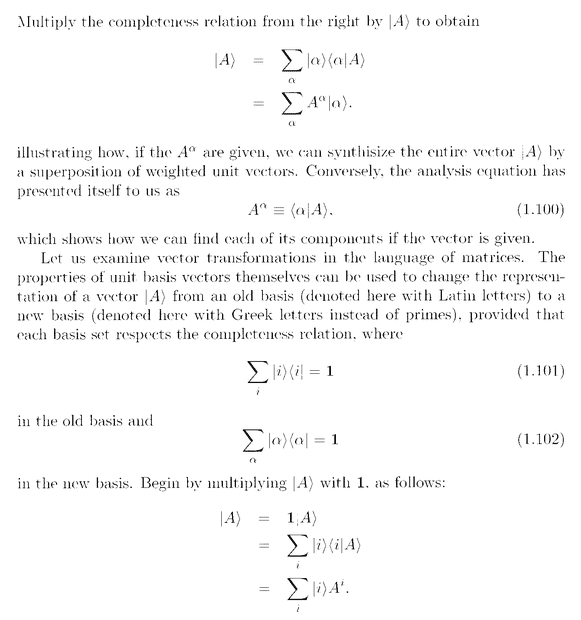Last edited:
andrewkirk said:
Hello Peter.
Broadly you have the correct idea but your approach relies on two implicit assumptions that do not hold in the general case.
Firstly, by writing ##\langle \alpha|## and ##|\alpha\rangle## as each having three components, you are assuming the vector space is finite dimensional. Dirac's notation is most used in quantum mechanics, where the vector spaces are usually infinite-dimensional, so such a representation as a finite set of components does not appear.
Secondly, you replace the inner product ##\langle\alpha|A\rangle## by a simple multiplication of a row vector ##\langle\alpha|## by a column vector ##|A\rangle##. That is only correct when those vectors are representations in an orthonormal basis. From your first embedded image, that seems to be the case here, but you need to exercise care that it will not always be the case. The more general formula requires use of a metric tensor so that ##\langle\alpha|A\rangle = \langle\alpha| \times M \times |\alpha\rangle## where ##M## is the representation of the metric tensor in the given basis. For an orthonormal basis ##M## will be the identity matrix (with an infinite number of rows and columns if the space is infinite-dimensional!) so the calculation collapses to look like what you have shown.
Lastly, to avoid confusion, avoid writing things like $$\vert A \rangle = \begin{bmatrix} A^1 \\ A^2 \\ A^3 \end{bmatrix}$$
The left-hand side is a vector in the space. The right-hand side is a representation of that vector in a particular basis. The two sides are not the same sort of object and should not be connected by an equals sign.

This can seem confusing if you've been introduced to linear algebra through vectors and matrices that are rows, columns or rectangle of numbers. Vector spaces are abstract objects, of which numeric number tuples are only one type.

andrewkirk said:
Hello Peter.
Broadly you have the correct idea but your approach relies on two implicit assumptions that do not hold in the general case.
Firstly, by writing ##\langle \alpha|## and ##|\alpha\rangle## as each having three components, you are assuming the vector space is finite dimensional. Dirac's notation is most used in quantum mechanics, where the vector spaces are usually infinite-dimensional, so such a representation as a finite set of components does not appear.
Secondly, you replace the inner product ##\langle\alpha|A\rangle## by a simple multiplication of a row vector ##\langle\alpha|## by a column vector ##|A\rangle##. That is only correct when those vectors are representations in an orthonormal basis. From your first embedded image, that seems to be the case here, but you need to exercise care that it will not always be the case. The more general formula requires use of a metric tensor so that ##\langle\alpha|A\rangle = \langle\alpha| \times M \times |\alpha\rangle## where ##M## is the representation of the metric tensor in the given basis. For an orthonormal basis ##M## will be the identity matrix (with an infinite number of rows and columns if the space is infinite-dimensional!) so the calculation collapses to look like what you have shown.
Lastly, to avoid confusion, avoid writing things like $$\vert A \rangle = \begin{bmatrix} A^1 \\ A^2 \\ A^3 \end{bmatrix}$$
The left-hand side is a vector in the space. The right-hand side is a representation of that vector in a particular basis. The two sides are not the same sort of object and should not be connected by an equals sign.

This can seem confusing if you've been introduced to linear algebra through vectors and matrices that are rows, columns or rectangle of numbers. Vector spaces are abstract objects, of which numeric number tuples are only one type.

andrewkirk said:
Hello Peter.
Broadly you have the correct idea but your approach relies on two implicit assumptions that do not hold in the general case.
Firstly, by writing ##\langle \alpha|## and ##|\alpha\rangle## as each having three components, you are assuming the vector space is finite dimensional. Dirac's notation is most used in quantum mechanics, where the vector spaces are usually infinite-dimensional, so such a representation as a finite set of components does not appear.
Secondly, you replace the inner product ##\langle\alpha|A\rangle## by a simple multiplication of a row vector ##\langle\alpha|## by a column vector ##|A\rangle##. That is only correct when those vectors are representations in an orthonormal basis. From your first embedded image, that seems to be the case here, but you need to exercise care that it will not always be the case. The more general formula requires use of a metric tensor so that ##\langle\alpha|A\rangle = \langle\alpha| \times M \times |\alpha\rangle## where ##M## is the representation of the metric tensor in the given basis. For an orthonormal basis ##M## will be the identity matrix (with an infinite number of rows and columns if the space is infinite-dimensional!) so the calculation collapses to look like what you have shown.
Lastly, to avoid confusion, avoid writing things like $$\vert A \rangle = \begin{bmatrix} A^1 \\ A^2 \\ A^3 \end{bmatrix}$$
The left-hand side is a vector in the space. The right-hand side is a representation of that vector in a particular basis. The two sides are not the same sort of object and should not be connected by an equals sign.

This can seem confusing if you've been introduced to linear algebra through vectors and matrices that are rows, columns or rectangle of numbers. Vector spaces are abstract objects, of which numeric number tuples are only one type.

DrClaude said:
To add to @andrewkirk answer, not that what you wrote for the bra is incorrect, it should read
## \langle \alpha | = \begin{bmatrix}
\bar{\alpha}^1 & \bar{\alpha}^2 & \bar{\alpha}^3
\end{bmatrix}
##
i.e., the vector components of the bra are the complex conjugates of those of the ket.
Thanks DrClaude ... appreciate your help ...

Peter

In the following I write ##a## instead of ##\alpha## cos it's quicker to type.

Peter, I find it more intuitive to write ##\sum_a |a\rangle\langle a | A\rangle## as
##\sum_a \langle a | A\rangle |a\rangle## because the first factor ##\langle a | A\rangle## in the summand is a scalar and the second is a vector, and we usually do scalar multiplication of vectors on the left.
That formula gives the projection of vector ##|A\rangle## onto the subspace generated by vector ##|a\rangle##.
You may recall from your linear algebra studies that, given a basis for a vector space, we can write any vector as the sum of its projections onto the subspaces generated by each of the basis vectors. Those projections are all orthogonal. We get the sum of those projections by summing over the basis vectors ##|a\rangle##. You can think of ##a## as being the label or index of the vector ##|a\rangle## - that just prevents us from having to write the summing subscript as ##|a\rangle## instead of ##a## which would be just a bit too notation-heavy - ie we can write ##\sum_a## instead of ##\sum{|a\rangle}##.

Summary:
Given a basis whose vectors correspond to a set of labels (also called an "index set") ##\mathscr B##, and a vector ##|A\rangle##, the projection of that vector on a vector ##|a\rangle## for ##a\in\mathscr B## is given by ##\langle a | A\rangle\ |a\rangle##. It follows by the definition of a basis that we can write a vector as the sum of its components in that basis, thus:
$$|A\rangle = \sum_{a\in\mathscr B} \langle a | A\rangle\ |a\rangle$$
For brevity one usually replaces the summation subscript ##a\in\mathscr B## by just ##a## to give the ##\sum_a## the text has.

•Math Amateur and topsquark
Re your question about the texts, I looked back at my copies of those two.
They both have exercises. The French one has more exercises than Shankar. OTOH Shankar provides solutions to some of the exercises, whereas the French one does not.
Some on this forum sing the praises of a QM text by Ballentine very highly. I have not read it myself, but I recall noting it, because I respect the opinions of those who were singing its praises.

•topsquark
Thanks Andrew ...

Reflecting on what you have written ...

Thanks again,

Peter